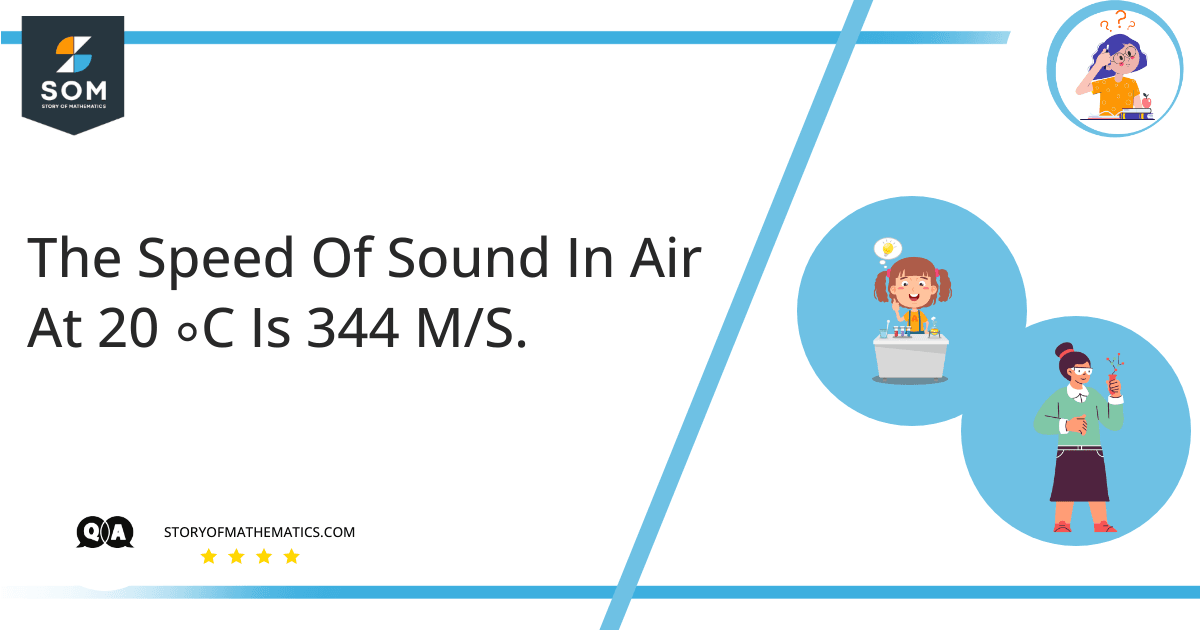# The speed of sound in air at 20 C is 344 m/s– In milliseconds, how long does it take for a sound wave to vibrate at a frequency at 784 Hz, or the pitch of the G5 on a piano?

– What’s the wavelength of an acoustic source one octave greater than the uppermost note?

The main objective of this question is to calculate the time required for  a sound wave to vibrate at a given frequency  and the wavelength of an acoustic source.

This question uses the concept of wavelength, frequency and speed of the wave. The distance among identical locations in adjacent phases of a waveform pattern carried in air or via a wire is defined as its wavelength and frequency is defined as reciprocal of time period.

a) We know that:

$\space v \space = \space f \space . \space \lambda$

And:

$\space T \space = \space \frac{1}{f}$

Given that:

$\space f_1 \space = \space 784 Hz$

$\space v \space = \space 344 \frac{m}{s}$

By putting values, we get:

$\space 344 \frac {m}{s} \space = \space (784 s^{-1}) \lambda_1$

By  simplifying, we get:

$\space \lambda_1 \space = \space 0.439 m$

The time period is given as:

$\space T_1 \space = \space \frac{1}{784}$

$\space T_1 \space = \space 1.28 \space \times \space 10^{-3}$

$\space T_1 \space = \space 1.28$

b) The wavelength of an acoustic source one octave greater than the uppermost note is calculated as:

$\space f_2 \space = \space 2 \space \times \space f_1$

By putting values, we get:

$\space = \space 2 \space \times \space 784$

$\space = \space 1568 hz$

Now:

$\space 344 \frac {m}{s} \space = \space (1568 s^{-1}) \lambda_2$

By  simplifying, we get:

$\space \lambda_2 \space = \space 0.219 m$

## Numerical Results

The time required for a sound wave to vibrate at a given frequency is:

$\space T_1 \space = \space 1.28$

The wavelength is:

$\space \lambda_2 \space = \space 0.219 m$

## Example

In milliseconds, how long does it take for a sound wave to vibrate at a frequency at $800 Hz$ when the sound speed is 344 \frac{m}{s} at 20 C \{circ} in air. What’s the wavelength of an acoustic source one octave greater than the uppermost note?

We know that:

$\space v \space = \space f \space . \space \lambda$

And:

$\space T \space = \space \frac{1}{f}$

Given that:

$\space f_1 \space = \space 800 Hz$

$\space v \space = \space 344 \frac{m}{s}$

By putting values, we get:

$\space 344 \frac {m}{s} \space = \space (800 s^{-1}) \lambda_1$

By  simplifying, we get:

$\space \lambda_1 \space = \space 0.43 m$

The time period is given as:

$\space T_1 \space = \space \frac{1}{784}$

$\space T_1 \space = \space 1.28 \space \times \space 10^{-3}$

$\space T_1 \space = \space 1.28$

Now the wavelength of an acoustic source one octave greater than the uppermost note is calculated as:

$\space f_2 \space = \space 2 \space \times \space f_1$

By putting values, we get:

$\space = \space 2 \space \times \space 784$

$\space = \space 1568 hz$

Now:

$\space 344 \frac {m}{s} \space = \space (1568 s^{-1}) \lambda_2$

By simplifying, we get:

$\space \lambda_2 \space = \space 0.219 m$